Courses

# Nuclear Physics Nat Level – 1

## 10 Questions MCQ Test Topic wise Tests for IIT JAM Physics | Nuclear Physics Nat Level – 1

Description
This mock test of Nuclear Physics Nat Level – 1 for IIT JAM helps you for every IIT JAM entrance exam. This contains 10 Multiple Choice Questions for IIT JAM Nuclear Physics Nat Level – 1 (mcq) to study with solutions a complete question bank. The solved questions answers in this Nuclear Physics Nat Level – 1 quiz give you a good mix of easy questions and tough questions. IIT JAM students definitely take this Nuclear Physics Nat Level – 1 exercise for a better result in the exam. You can find other Nuclear Physics Nat Level – 1 extra questions, long questions & short questions for IIT JAM on EduRev as well by searching above.
*Answer can only contain numeric values
QUESTION: 1

### The half life of a radioactive substance is 3h and its activity is 1µCi. Then the activity after 9h will be (in µCi)

Solution: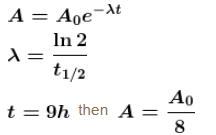*Answer can only contain numeric values
QUESTION: 2

### An electron positron pair (rest mass of each particle = 0.51 MeV) is produced by a γ -ray photon of energy 2.42 MeV. The K.E. (in MeV) imparted to each of the charged particles shall be :

Solution:

Rest mass energy + kinetic energy = energy of gamma photon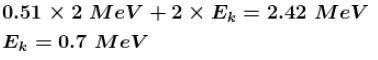*Answer can only contain numeric values
QUESTION: 3

### The probability of a radioactive atoms to survive 5 times longer than its half value period is.

Solution:

Decay period = Half life × No. of half lives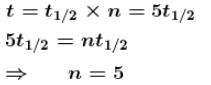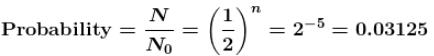*Answer can only contain numeric values
QUESTION: 4

A radioactive nuclie decay as follows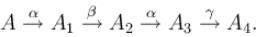If mass number and atomic number of A are 180 and 72 respectively then atomic number for A4 will be.

Solution:

72A180 -α->70A1176 -β->71A2176 -α->69A3172-γ->69A4174

*Answer can only contain numeric values
QUESTION: 5

After emission of an α -particle by a radioactive element 84X212, the resulting mass number would be.

Solution: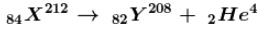*Answer can only contain numeric values
QUESTION: 6

If the mass of proton = 1.008 amu and mass of neutron = 1.009 amu then binding energy (in MeV) per nucleon for 4Be9  (mass = 9.012 amu) would be :

Solution: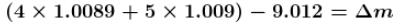Binding energy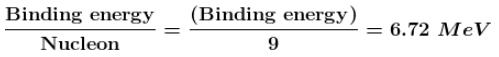*Answer can only contain numeric values
QUESTION: 7

After a time equal to four half lives, the percentage of radioactive material remaining undecayed is :

Solution: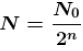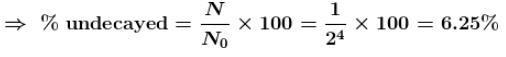*Answer can only contain numeric values
QUESTION: 8

The half-life of cobalt 60 is 5.25 years. How long after a new sample is delivered, will the activity have decreased to about one third (1/3) of its original value. (Provide the time in years).

Solution: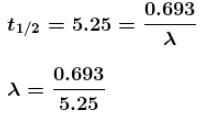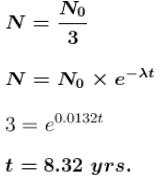*Answer can only contain numeric values
QUESTION: 9

The mean life of a radioactive sample is 100 years. Then after 100 years, what percentage of the sample remains active.

Solution: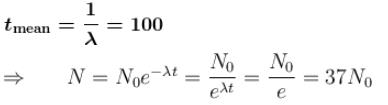*Answer can only contain numeric values
QUESTION: 10

1014 fissions per second are taking place in a nuclear reactor having efficiency 40%. The energy released per fission is 250 MeV. The power output (in Watts) of the reactor is :

Solution:

Power output 0.4 x power input = 0.4 x 250 x 106 x 1.6 x 10-19 x 1014 = 1600W.